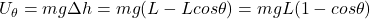## A pendulum consists of a massless stiff rod of length L hanging from a nearly frictionless axle, with a mass m at the end of the rod. Calcul

Question

A pendulum consists of a massless stiff rod of length L hanging from a nearly frictionless axle, with a mass m at the end of the rod. Calculate the gravitational potential energy as a function of the angle, θ, measured from the vertical. Set U = 0 at the location of the mass when the pendulum is hanging straight down.

in progress 0
6 months 2021-07-29T02:19:55+00:00 1 Answers 22 views 0

mgL(1 – cosθ)

Explanation:

At angle θ, the vertical distance from the mass m to the pivotal axle is Lcosθ. While at U=0, this distance is L. Due to the mass is hanging straight down. Therefore the vertical distance from this lowest point to point at angle θ is

L – Lcosθ

The change in potential energy would be this height difference times mgwhere g is the gravitational constant